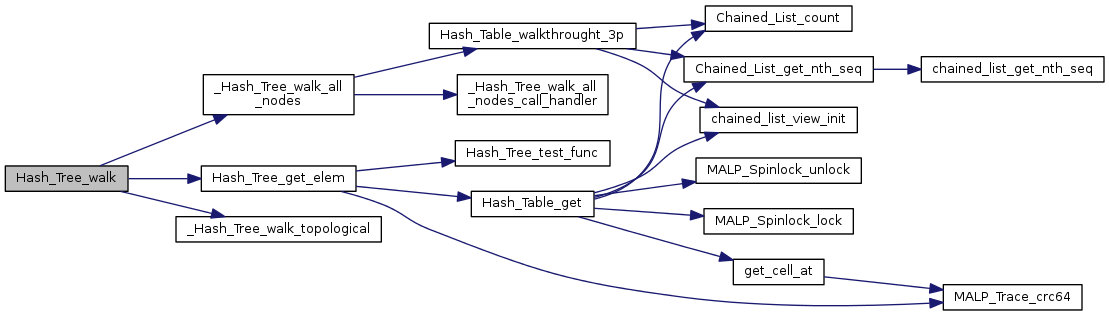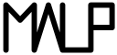Multi-ApplicationOnlineProfiling  2.1
Hash_Tree.c File Reference
`#include "Hash_Tree.h"`
`#include "Debug.h"`
`#include "CRC64.h"`
Include dependency graph for Hash_Tree.c: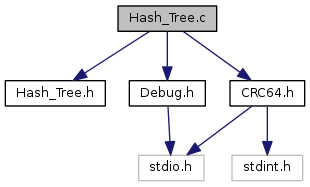Go to the source code of this file.

## Functions

void Hash_Tree_node_init (struct Hash_Tree_node *node, struct Hash_Tree_node *parent, void *request, size_t request_size, void *payload, size_t payload_size)

void Hash_Tree_node_release (struct Hash_Tree_node *node)

void __Hash_Tree_node_release (void *node)

void Hash_Tree_node_push_child (struct Hash_Tree_node *node, struct Hash_Tree_node *child)

int Hash_Tree_test_func (void *elem, uint64_t key, void *arg)

void Hash_Tree_init (struct Hash_Tree *htr, size_t request_size, size_t payload_size)

void Hash_Tree_call_free (void *elem, void *pfree_func)

void Hash_Tree_release (struct Hash_Tree *htr, void(*free_func)(void *))

int Hash_Tree_elem_exits (struct Hash_Tree *htr, void *request)

struct Hash_Tree_node * Hash_Tree_get_elem (struct Hash_Tree *htr, void *request)

struct Hash_Tree_node * Hash_Tree_push (struct Hash_Tree *htr, void *parent_request, void *new_request, void *payload)

void _Hash_Tree_walk_all_nodes_call_handler (void *p_node, void *phandler, void *extra_arg)

void _Hash_Tree_walk_all_nodes (struct Hash_Tree *htr, void(*handler)(void *, void *), void *extra_arg)

void _Hash_Tree_walk_topological (struct Hash_Tree *htr, struct Hash_Tree_node *node, void(*handler)(void *, void *), void *extra_arg)

void Hash_Tree_walk (struct Hash_Tree *htr, void *starting_point_request, void(*handler)(void *, void *), void *extra_arg)

## Function Documentation

 void __Hash_Tree_node_release ( void * node )

Definition at line 74 of file Hash_Tree.c.

Here is the call graph for this function: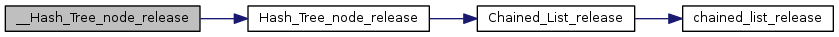Here is the caller graph for this function: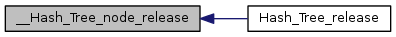void _Hash_Tree_walk_all_nodes ( struct Hash_Tree * htr, void(*)(void *, void *) handler, void * extra_arg )

Definition at line 227 of file Hash_Tree.c.

Here is the call graph for this function: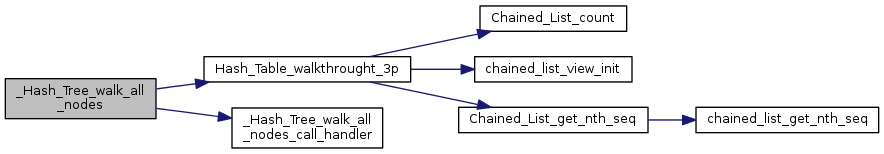Here is the caller graph for this function: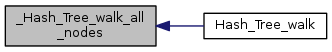void _Hash_Tree_walk_all_nodes_call_handler ( void * p_node, void * phandler, void * extra_arg )

Definition at line 215 of file Hash_Tree.c.

Here is the caller graph for this function: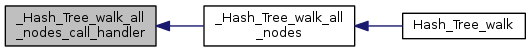void _Hash_Tree_walk_topological ( struct Hash_Tree * htr, struct Hash_Tree_node * node, void(*)(void *, void *) handler, void * extra_arg )

Definition at line 232 of file Hash_Tree.c.

Here is the caller graph for this function: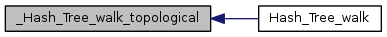void Hash_Tree_call_free ( void * elem, void * pfree_func )

Definition at line 133 of file Hash_Tree.c.

Here is the caller graph for this function: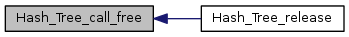int Hash_Tree_elem_exits ( struct Hash_Tree * htr, void * request )

Definition at line 152 of file Hash_Tree.c.

Here is the call graph for this function: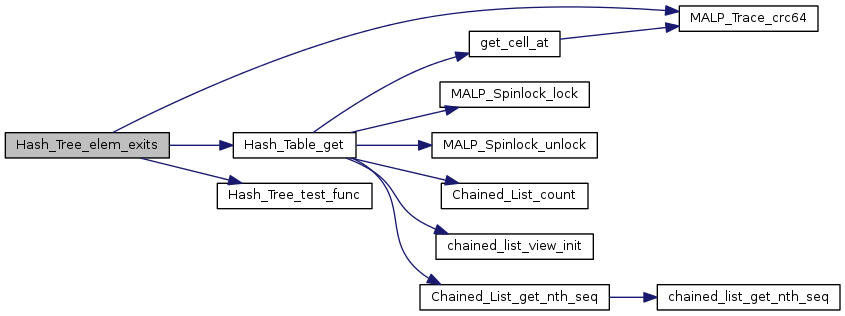struct Hash_Tree_node* Hash_Tree_get_elem ( struct Hash_Tree * htr, void * request )

Definition at line 158 of file Hash_Tree.c.

Here is the call graph for this function: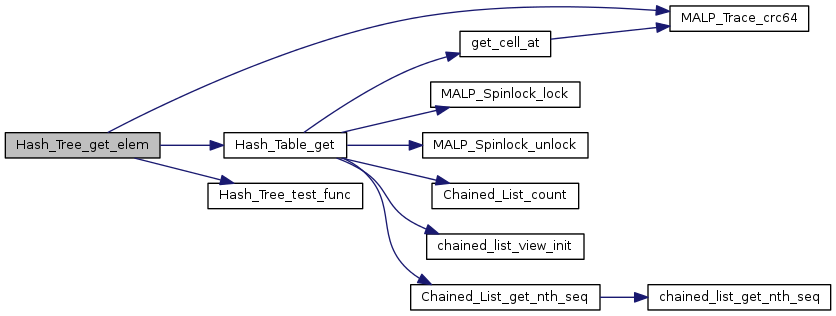Here is the caller graph for this function: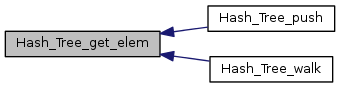void Hash_Tree_init ( struct Hash_Tree * htr, size_t request_size, size_t payload_size )

Definition at line 118 of file Hash_Tree.c.

Here is the call graph for this function: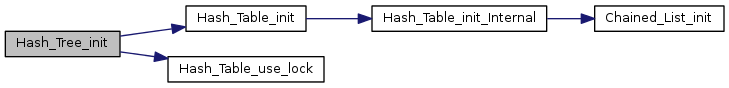void Hash_Tree_node_init ( struct Hash_Tree_node * node, struct Hash_Tree_node * parent, void * request, size_t request_size, void * payload, size_t payload_size )

Definition at line 28 of file Hash_Tree.c.

Here is the call graph for this function: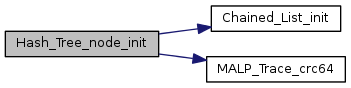Here is the caller graph for this function: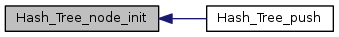void Hash_Tree_node_push_child ( struct Hash_Tree_node * node, struct Hash_Tree_node * child )

Definition at line 80 of file Hash_Tree.c.

Here is the call graph for this function:Here is the caller graph for this function: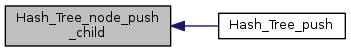void Hash_Tree_node_release ( struct Hash_Tree_node * node )

Definition at line 64 of file Hash_Tree.c.

Here is the call graph for this function: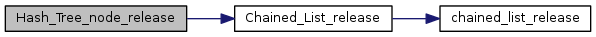Here is the caller graph for this function: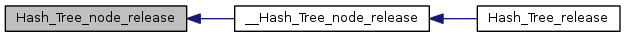struct Hash_Tree_node* Hash_Tree_push ( struct Hash_Tree * htr, void * parent_request, void * new_request, void * payload )

Definition at line 164 of file Hash_Tree.c.

Here is the call graph for this function: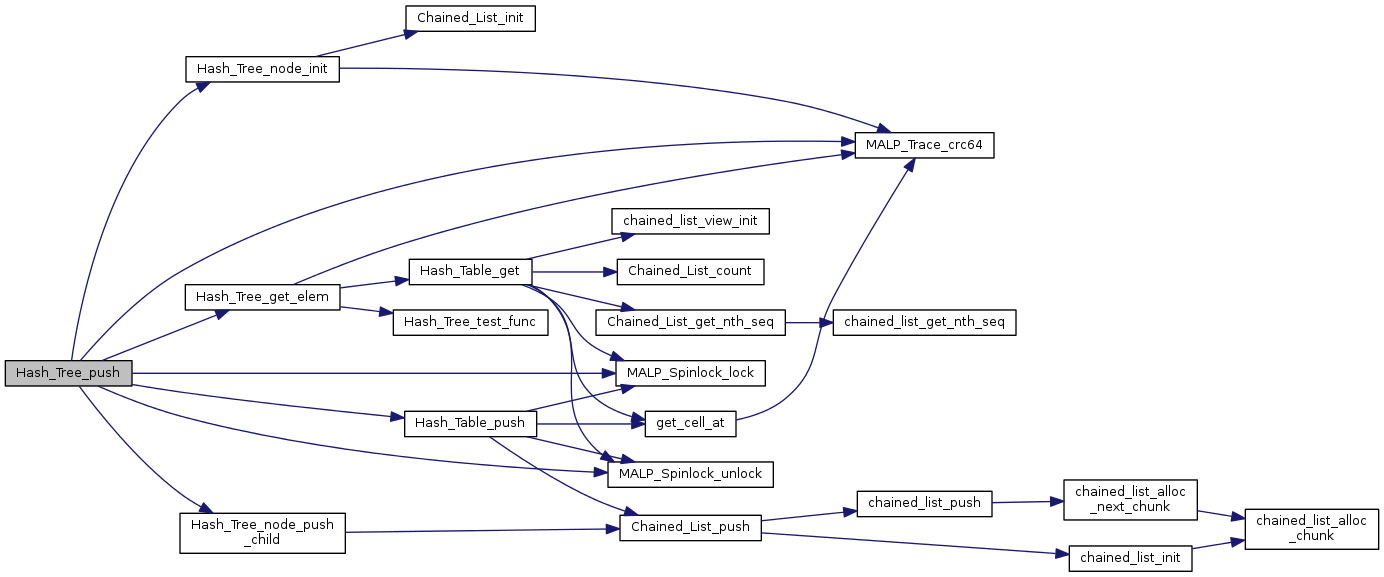void Hash_Tree_release ( struct Hash_Tree * htr, void(*)(void *) free_func )

Definition at line 143 of file Hash_Tree.c.

Here is the call graph for this function: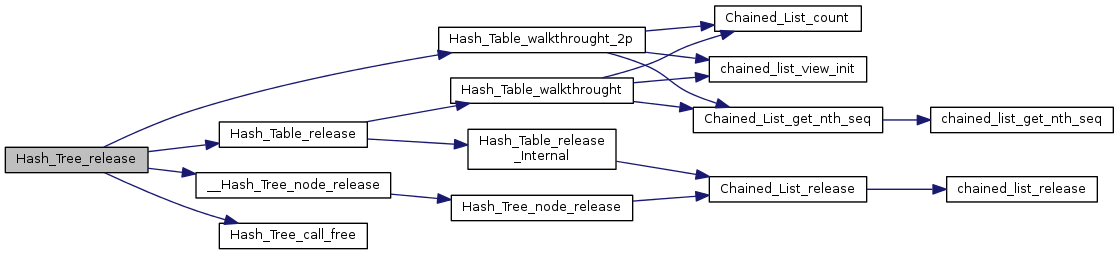int Hash_Tree_test_func ( void * elem, uint64_t key, void * arg )

Definition at line 91 of file Hash_Tree.c.

Here is the caller graph for this function: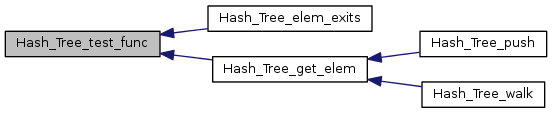void Hash_Tree_walk ( struct Hash_Tree * htr, void * starting_point_request, void(*)(void *, void *) handler, void * extra_arg )

Definition at line 252 of file Hash_Tree.c.

Here is the call graph for this function: